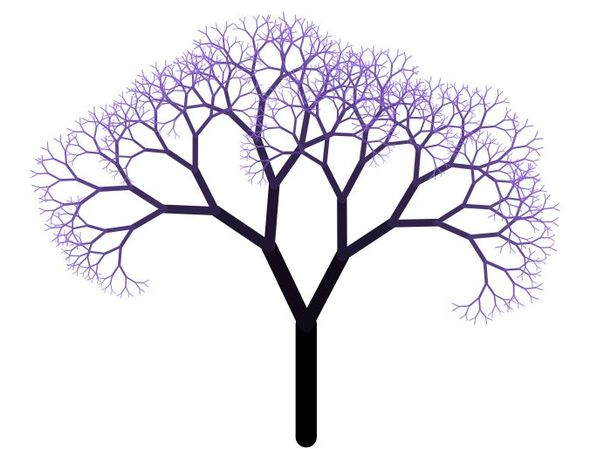Quiz 5: Trees and Transformations

[This quiz is due next Monday, September 17 at the beginning of class. Please remember to print out your solution and bring it to class! If you make a cool picture, go ahead and also post it on Piazza :-)]

A variety of natural phenomena exhibit self-similar or "fractal" structures, i.e., similar patterns that recur at progressively smaller scales:In computer graphics, one simple way to model fractal behavior is to recursively apply the types of transformations we've been studying in class (rotation, translation, scale, etc.) to some graphical primitive (like a line segment or rectangle). For this assignment, you will design a function that draws a fractal binary tree in 2D:Your task is to write a routine (in pseudocode) that (i) draws a single edge in the tree, and (ii) recursively draws the (smaller) left and right branches. The only argument this function needs to take is the current depth of the tree. When the depth reaches zero, recursion should terminate. Your challenge, then, is to apply the right transformations at just the right moment, so that the output looks something like the tree depicted above.

Your routine should have the following structure:

drawSubTree( depth )
{
if( depth == 0 ) return;

// draw some geometry for the current edge

// apply some transformations

drawSubTree( depth-1 );

// apply some transformations

drawSubTree( depth-1 );

// apply some transformations
}


As you can see, most of the routine has already been written for you. Your job is to fill in the part that says "draw a rectangle" and "apply some transformations." You should assume that the following pseudocode commands are available to you:

rotate( theta );    // rotate by an angle theta, in radians
translate( u, v );  // apply a horizontal translation u, and a vertical translation v
scale( s );         // uniformly scale by a factor s
rect( x, y, w, h ); // draw a rectangle with corner (x,y), width w, and height h


You also can assume that transformations are applied in the same way as in OpenGL: the last transformation gets applied first. For instance, if you write

rotate( theta );
translate( u, v );
rect( x, y, width, height );


then the rectangle will be translated then rotated. If you instead write

translate( u, v );
rotate( theta );
rect( x, y, width, height );


the rectangle will be rotated then translated. Note that you should not use the depth value to determine the width/height or position of the rectangle - everything should be done purely through the transformations.

Hint: Think very carefully about how transformations get applied as you move up and down the call stack. (Do you need to "undo" any transformations?)

# Optional: Play Around in HTML5 Canvas Sandbox

For the quiz, you don't have to write "real code"; just pseudocode. However, it can be hard to write correct pseudocode without a visualization of what you're writing. You may therefore find it very helpful - and fun! - to try it out in a real environment. We've created a sandbox for you to try out here:

HTML5 Canvas Sandbox for Tree Drawing

This sandbox allows you to call drawing and transformation routines using HTML5 Canvas, which is a modern standard for drawing 2D graphics in a web browser. Most of the code has been written for you already. The basic idea is that you have a context, called ctx, which can make all sorts of draw calls. For instance, to draw a rectangle you can write

ctx.beginPath();
ctx.rect(-10,-40,20,80); // the four arguments specify x, y, width, height
ctx.fill();


Geometric transformations look nearly identical to the pseuedocode commands given above:

ctx.rotate( theta ); // rotate by an angle theta, in radians
ctx.translate( u, v ); // apply a horizontal translation u, and a vertical translation v
ctx.scale( a, b ); // scale by a in the horizontal direction and b in the vertical direction
ctx.rect( x, y, w, h ); // draw a rectangle with corner (x,y), width w, and height h


As above, transformations get applied in the order "last transformation first." And again, you should think about how transformations should get applied and then reversed as you go up and down the call stack.

Of course, you're welcome to get as fancy as you like here; here's a reference guide for more functions in Canvas.

If you do use canvas for your solution please print out your drawing and turn it in! We want to see what cool stuff you make. We definitely encourage creativity here - try changing the shape, size, color, style of the tree---or even (if you're feeling ambitious) the branching factor. If you make something particularly cool, please post it on Piazza so that we can see it in its full-color glory.